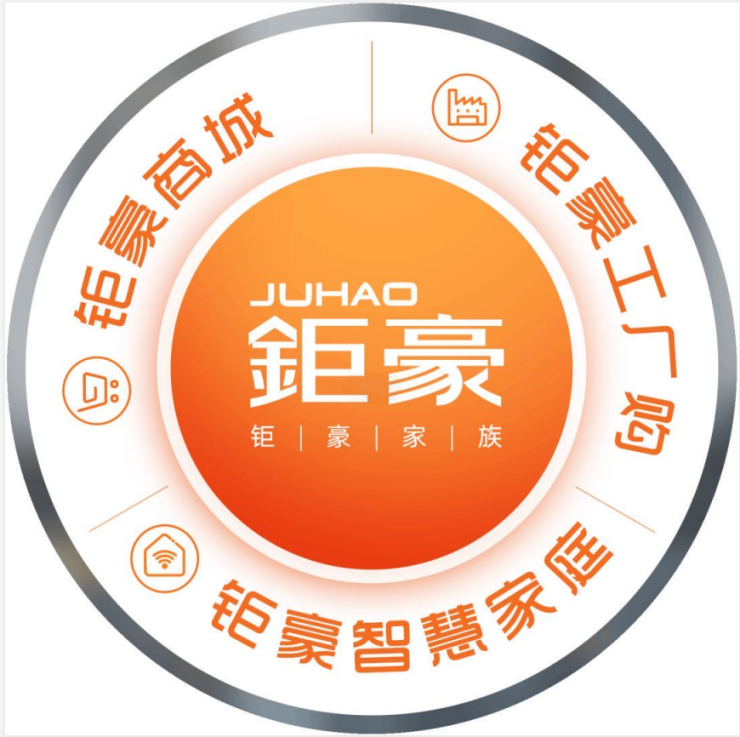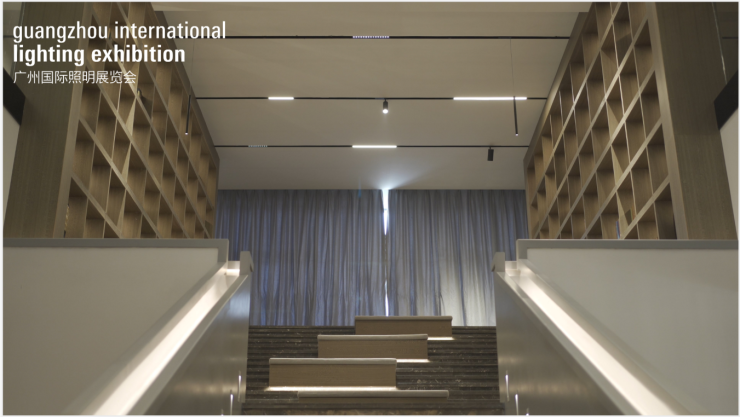|

# 首席对话 | 钜豪照明:三驾马车引领照明行业转型升级遍布全国数千家钜豪工厂购门店，并且拥有少有的单一品牌全品类灯饰照明交易平台的钜豪，2020年力推以PLC光控为核心的智慧家庭生态。创立23载的广东钜豪照明电器有限公司一直对市场需求保持高度敏锐的嗅觉，不断引领灯饰行业的创变方向，优质实现了传统灯饰企业向智能家居平台的转型升级。未来照明企业如何从激烈的市场竞争中脱颖而出，构建自己的商业壁垒?钜豪给出了清晰的答案。许建龙

钜豪集团董事长

把脉当下2020年的疫情黑天鹅对各行各业产生了深刻影响，灯饰照明行业也不例外，但钜豪是个例外。“疫情并没有对钜豪带来太大影响，反而给了我们充足的时间历练内功。”钜豪集团董事长许建龙表示，正是有了疫情年在设计与技术上沉淀，钜豪才能在今年3月中重磅推出以PLC光控为核心的智慧家庭生态。

所谓厚积薄发源于长时间的坚持与累积，推出智慧家庭之前，钜豪经历了长达45个月的潜心研发。作为一个有着二十多年市场实践经验的老牌企业，钜豪对市场有着清醒的认知。在5G、IoT、AI人工智能等技术的驱动下，智能家居成为泛家居行业的未来方向。深度研究市场之后，钜豪推出了独具特色的智慧家庭生态模式，该模式呈现4大优势：

1、软件系统优势

钜豪拥有自己的软硬件技术开发团队，拥有完全自主知识产权的SaaS系统，不仅可以与阿里华为等IoT平台合作，也形成了以光控为核心竞争力的智慧家庭生态链。

2、多平台链接

只要云对云打通，钜豪自有的智慧家庭系统就能接入阿里华为等多个平台的物联网体系，实现广泛兼容，以带给客户更好的体验。

3、PLC技术优势

钜豪智慧家庭系统采用行业先进的PLC技术，提升企业市场竞争力。PLC技术比传统的ZigBee、WIFI等技术更加稳定可靠，也能与WIFI、ZigBee、蓝牙等无线方案相融共生，实现无障碍互联互通。传统有线方案需要预埋线，主要针对前装市场，而钜豪的PLC技术也能服务后装市场，凭借技术上的优势与无线连接方式直接竞争。

4、生态链优势

为了更好地发挥智能生态的协同效应，为消费者提供更加便捷、人性化的智慧家庭解决方案，钜豪不仅将传统照明升级为“智光”，更自主研发了智能电工、安防监控、智能窗帘、智能门锁、智能晾晒等，共6大智能产品体系，全屋智能，不止于光。

许建龙表示，智慧家庭生态将成为钜豪未来5-10年的战略方向。未来1年，钜豪将扶持建设200家智慧家庭体验馆，销售超过100万个设备端口，并建立完整的生态伙伴，加速灯饰照明行业往智能化、数字化方向转型。100平方米门店承载10000平方米的产品，自建商城平台、发力工厂购模式，多渠道多通路全方位赋能经销商

尽管线上电商不断冲击线下产业，对于重体验的家居行业来说，线下门店依然是品牌发展最重要的渠道。如何在新形势下更多地赋能渠道，帮助经销商屹立于市场，实现品牌健康持久的发展，成为考验泛家居品牌的新命题。

为了提高经销商的抗风险能力与盈利能力，钜豪从2016年开始自建全品类灯饰照明交易平台的钜豪商城。钜豪商城拥有二十多种大类数万款灯饰照明产品，门店可以通过终端机连接钜豪商城，让100平方米门店承载10000平方米的产品。通过配灯大师、找我家自动生成效果图、线上支付等自主采购线上工具，为客户创造更多价值，帮助门店提高坪效，实现更加轻松的营销。与此同时，钜豪商城还拥有强大的分销系统，借助移动电商为经销商引流。“以前县市级市场只能开实体门店，现在可以采用更加灵活高效的分销方式，打破传统门店的限制。比如可以在五金店、物业管理处等场景植入钜豪分销系统，形成强大的引流效应，为经销商赋能。”许建龙说。除了用商城赋能门店，钜豪还大力发展终端“工厂购”模式，打造灯饰照明界的“奥特莱斯”，为经销商创造更多的盈利点。钜豪“工厂购”模式依托于钜豪工业园逾二万平方的旗舰店，为经销商在别墅及高端定制等方面提供坚实的保障，并通过大型团购、爆破营销、线上直播等方式引爆终端。

除了零售渠道，钜豪还积极开拓房地产商、渠道品牌、装修装饰公司等集采工程渠道。在淘宝、天猫、拼多多等传统电商之外，还不断深耕阿里智能、华为商城、抖音等渠道，通过多渠道、多通路全方位赋能经销商。

与此同时，为了让经销商更好地适应新的市场形势，尤其是智能时代带来的一系列变化，钜豪还在全国各地建立省级服务中心，对门店人员的营销、设计、服务等综合能力进行培训和指导，帮助门店更好地服务客户，获得市场竞争优势。

赢领未来从1998年开始做艺术灯，到如今做智能家居，许建龙感慨万千。“灯饰行业与服装行业有类似性，都有潮流感，产品很容易被市场淘汰。灯饰行业门槛低、市场竞争激烈，资本、IPO企业越来越强，对于灯饰企业来说，未来之路将更加艰辛。”他认为，未来灯饰行业的发展模式有两条路子：要么做专做强，将专业生产做到酣畅淋漓;要么走向智能生态链，形成自己的商业壁垒。

在专业生产方面，许建龙认为，“健康光”、“智光”、“结构化”是未来灯饰照明的三大趋势。比起灯，未来人们将更注重光为生活带来的改变。后疫情时代，人们居住空间对健康提出了更高要求，不伤眼、高显指(低于显指<80的灯光属于污染光)等属性的“健康光”呼声会越来越高。5G技术加速了智能时代的到来，灯饰照明如何融入智能场景?将是值得企业好好思考的问题。他强调“智光”不仅是要健康，还要足够聪明，能够真正让消费者的生活变得更加便利舒适，而不是为生活添堵。另外，许建龙认为，结构化、多功能也是值得关注的方向，未来灯饰设计可能走向模块化。“灯具可以像变形金刚一样，可以随意搭配、自由组合。

在生态链方面，许建龙认为PLC技术的落地是行业的分水岭，将很大程度改变智能家居生态，未来会成为智能家居的主流通讯协议。华为主推PLC通信技术，一定程度反映了这种趋势。许建龙表示，钜豪从2017年开始试水智能家居，到2021年才宣布推出智慧家庭，一方面是为了夯实技术基础，另一方面也是为了重点发力PLC技术。在PLC通讯落地之前，大众市场都在做ZigBee、WIFI方案，无线技术相对稳定度不高，当稳定性超出一定程度就会变“智障”，极大影响消费体验，用许建龙的话说技术“底盘不够结实”，也影响了智能家居的推广与应用。而“PLC+无线”的方案能够彻底解决这个问题，对构建智慧家庭生态更有优势，可以预见，随着PLC技术的广泛应用，将带动智能家居飞速发展。

建言GILE

作为行业规模较大的灯饰照明盛会，GILE借助展会平台的优势大力推动了行业发展，这么多年来钜豪跟随光亚展共同成长。GILE是照明企业宣传企业、推广产品的优选平台，也是较新行业趋势的发布平台，随着灯饰照明行业不断升级优化，我们非常期待GILE能够继续引领智能化的趋势，引领行业走向新的发展格局。企业名片：广东钜豪照明电器有限公司

展位号：9.2 C28

广东钜豪照明创立于1998年，经过23年的发展，已经成为灯饰照明以及智能家居行业的品牌企业。钜豪在中国灯饰制造基地广东中山横栏拥有面积超100亩的工业园区。钜豪通过了ISO9000质量管理体系认证，先后荣获“国家免检产品”、“广东省著名商标”、“中国驰名商标”、“国家高新技术企业”“广东省名牌产品”国家质检总局质量抽查连续六年合格企业等荣誉称号，并且连续11年被评为广东省守合同重信用企业。钜豪还是豪华星级酒店的优选合作品牌，钜豪的产品不仅服务于香格里拉大酒店、希尔顿酒店、威斯汀酒店等星级酒店，并且点亮了北京人民大会堂。同时钜豪智慧家庭与金螳螂达成战略合作，并将钜豪智能方案广泛应用于其高档装修项目。钜豪IOT-P混联技术，率先制定PLC光控与WIFI互联创新解决方案。物联网时代，致力于应用较新科技，提供行业先进的健康光、智能光、艺术光一站式服务。全屋智能，不止于光。构建阿里云、华为生态朋友圈，智能跨越，相融共生，重新开启智能家居技术与商业发展新征程!

`声明：本文由入驻焦点开放平台的作者撰写，除焦点官方账号外，观点仅代表作者本人，不代表焦点立场错误信息举报电话： 400-099-0099，邮箱：jubao@vip.sohu.com，或点此进行意见反馈，或点此进行举报投诉。`A B C D E F G H J K L M N P Q R S T W X Y Z
A - B - C - D - E
• A
• 鞍山
• 安庆
• 安阳
• 安顺
• 安康
• 澳门
• B
• 北京
• 保定
• 包头
• 巴彦淖尔
• 本溪
• 蚌埠
• 亳州
• 滨州
• 北海
• 百色
• 巴中
• 毕节
• 保山
• 宝鸡
• 白银
• 巴州
• C
• 承德
• 沧州
• 长治
• 赤峰
• 朝阳
• 长春
• 常州
• 滁州
• 池州
• 长沙
• 常德
• 郴州
• 潮州
• 崇左
• 重庆
• 成都
• 楚雄
• 昌都
• 慈溪
• 常熟
• D
• 大同
• 大连
• 丹东
• 大庆
• 东营
• 德州
• 东莞
• 德阳
• 达州
• 大理
• 德宏
• 定西
• 儋州
• 东平
• E
• 鄂尔多斯
• 鄂州
• 恩施
F - G - H - I - J
• F
• 抚顺
• 阜新
• 阜阳
• 福州
• 抚州
• 佛山
• 防城港
• G
• 赣州
• 广州
• 桂林
• 贵港
• 广元
• 广安
• 贵阳
• 固原
• H
• 邯郸
• 衡水
• 呼和浩特
• 呼伦贝尔
• 葫芦岛
• 哈尔滨
• 黑河
• 淮安
• 杭州
• 湖州
• 合肥
• 淮南
• 淮北
• 黄山
• 菏泽
• 鹤壁
• 黄石
• 黄冈
• 衡阳
• 怀化
• 惠州
• 河源
• 贺州
• 河池
• 海口
• 红河
• 汉中
• 海东
• 怀来
• I
• J
• 晋中
• 锦州
• 吉林
• 鸡西
• 佳木斯
• 嘉兴
• 金华
• 景德镇
• 九江
• 吉安
• 济南
• 济宁
• 焦作
• 荆门
• 荆州
• 江门
• 揭阳
• 金昌
• 酒泉
• 嘉峪关
K - L - M - N - P
• K
• 开封
• 昆明
• 昆山
• L
• 廊坊
• 临汾
• 辽阳
• 连云港
• 丽水
• 六安
• 龙岩
• 莱芜
• 临沂
• 聊城
• 洛阳
• 漯河
• 娄底
• 柳州
• 来宾
• 泸州
• 乐山
• 六盘水
• 丽江
• 临沧
• 拉萨
• 林芝
• 兰州
• 陇南
• M
• 牡丹江
• 马鞍山
• 茂名
• 梅州
• 绵阳
• 眉山
• N
• 南京
• 南通
• 宁波
• 南平
• 宁德
• 南昌
• 南阳
• 南宁
• 内江
• 南充
• P
• 盘锦
• 莆田
• 平顶山
• 濮阳
• 攀枝花
• 普洱
• 平凉
Q - R - S - T - W
• Q
• 秦皇岛
• 齐齐哈尔
• 衢州
• 泉州
• 青岛
• 清远
• 钦州
• 黔南
• 曲靖
• 庆阳
• R
• 日照
• 日喀则
• S
• 石家庄
• 沈阳
• 双鸭山
• 绥化
• 上海
• 苏州
• 宿迁
• 绍兴
• 宿州
• 三明
• 上饶
• 三门峡
• 商丘
• 十堰
• 随州
• 邵阳
• 韶关
• 深圳
• 汕头
• 汕尾
• 三亚
• 三沙
• 遂宁
• 山南
• 商洛
• 石嘴山
• T
• 天津
• 唐山
• 太原
• 通辽
• 铁岭
• 泰州
• 台州
• 铜陵
• 泰安
• 铜仁
• 铜川
• 天水
• 天门
• W
• 乌海
• 乌兰察布
• 无锡
• 温州
• 芜湖
• 潍坊
• 威海
• 武汉
• 梧州
• 渭南
• 武威
• 吴忠
• 乌鲁木齐
X - Y - Z
• X
• 邢台
• 徐州
• 宣城
• 厦门
• 新乡
• 许昌
• 信阳
• 襄阳
• 孝感
• 咸宁
• 湘潭
• 湘西
• 西双版纳
• 西安
• 咸阳
• 西宁
• 仙桃
• 西昌
• Y
• 阳泉
• 运城
• 营口
• 盐城
• 扬州
• 鹰潭
• 宜春
• 烟台
• 宜昌
• 岳阳
• 益阳
• 永州
• 阳江
• 云浮
• 玉林
• 宜宾
• 雅安
• 玉溪
• 延安
• 榆林
• 银川
• Z
• 张家口
• 镇江
• 舟山
• 漳州
• 淄博
• 枣庄
• 郑州
• 周口
• 驻马店
• 株洲
• 张家界
• 珠海
• 湛江
• 肇庆
• 中山
• 自贡
• 资阳
• 遵义
• 昭通
• 张掖
• 中卫

1室1厅1厨1卫1阳台

1
2
3
4
5

0
1
2

1

1

0
1
2
3报名成功，资料已提交审核A B C D E F G H J K L M N P Q R S T W X Y Z
A - B - C - D - E
• A
• 鞍山
• 安庆
• 安阳
• 安顺
• 安康
• 澳门
• B
• 北京
• 保定
• 包头
• 巴彦淖尔
• 本溪
• 蚌埠
• 亳州
• 滨州
• 北海
• 百色
• 巴中
• 毕节
• 保山
• 宝鸡
• 白银
• 巴州
• C
• 承德
• 沧州
• 长治
• 赤峰
• 朝阳
• 长春
• 常州
• 滁州
• 池州
• 长沙
• 常德
• 郴州
• 潮州
• 崇左
• 重庆
• 成都
• 楚雄
• 昌都
• 慈溪
• 常熟
• D
• 大同
• 大连
• 丹东
• 大庆
• 东营
• 德州
• 东莞
• 德阳
• 达州
• 大理
• 德宏
• 定西
• 儋州
• 东平
• E
• 鄂尔多斯
• 鄂州
• 恩施
F - G - H - I - J
• F
• 抚顺
• 阜新
• 阜阳
• 福州
• 抚州
• 佛山
• 防城港
• G
• 赣州
• 广州
• 桂林
• 贵港
• 广元
• 广安
• 贵阳
• 固原
• H
• 邯郸
• 衡水
• 呼和浩特
• 呼伦贝尔
• 葫芦岛
• 哈尔滨
• 黑河
• 淮安
• 杭州
• 湖州
• 合肥
• 淮南
• 淮北
• 黄山
• 菏泽
• 鹤壁
• 黄石
• 黄冈
• 衡阳
• 怀化
• 惠州
• 河源
• 贺州
• 河池
• 海口
• 红河
• 汉中
• 海东
• 怀来
• I
• J
• 晋中
• 锦州
• 吉林
• 鸡西
• 佳木斯
• 嘉兴
• 金华
• 景德镇
• 九江
• 吉安
• 济南
• 济宁
• 焦作
• 荆门
• 荆州
• 江门
• 揭阳
• 金昌
• 酒泉
• 嘉峪关
K - L - M - N - P
• K
• 开封
• 昆明
• 昆山
• L
• 廊坊
• 临汾
• 辽阳
• 连云港
• 丽水
• 六安
• 龙岩
• 莱芜
• 临沂
• 聊城
• 洛阳
• 漯河
• 娄底
• 柳州
• 来宾
• 泸州
• 乐山
• 六盘水
• 丽江
• 临沧
• 拉萨
• 林芝
• 兰州
• 陇南
• M
• 牡丹江
• 马鞍山
• 茂名
• 梅州
• 绵阳
• 眉山
• N
• 南京
• 南通
• 宁波
• 南平
• 宁德
• 南昌
• 南阳
• 南宁
• 内江
• 南充
• P
• 盘锦
• 莆田
• 平顶山
• 濮阳
• 攀枝花
• 普洱
• 平凉
Q - R - S - T - W
• Q
• 秦皇岛
• 齐齐哈尔
• 衢州
• 泉州
• 青岛
• 清远
• 钦州
• 黔南
• 曲靖
• 庆阳
• R
• 日照
• 日喀则
• S
• 石家庄
• 沈阳
• 双鸭山
• 绥化
• 上海
• 苏州
• 宿迁
• 绍兴
• 宿州
• 三明
• 上饶
• 三门峡
• 商丘
• 十堰
• 随州
• 邵阳
• 韶关
• 深圳
• 汕头
• 汕尾
• 三亚
• 三沙
• 遂宁
• 山南
• 商洛
• 石嘴山
• T
• 天津
• 唐山
• 太原
• 通辽
• 铁岭
• 泰州
• 台州
• 铜陵
• 泰安
• 铜仁
• 铜川
• 天水
• 天门
• W
• 乌海
• 乌兰察布
• 无锡
• 温州
• 芜湖
• 潍坊
• 威海
• 武汉
• 梧州
• 渭南
• 武威
• 吴忠
• 乌鲁木齐
X - Y - Z
• X
• 邢台
• 徐州
• 宣城
• 厦门
• 新乡
• 许昌
• 信阳
• 襄阳
• 孝感
• 咸宁
• 湘潭
• 湘西
• 西双版纳
• 西安
• 咸阳
• 西宁
• 仙桃
• 西昌
• Y
• 阳泉
• 运城
• 营口
• 盐城
• 扬州
• 鹰潭
• 宜春
• 烟台
• 宜昌
• 岳阳
• 益阳
• 永州
• 阳江
• 云浮
• 玉林
• 宜宾
• 雅安
• 玉溪
• 延安
• 榆林
• 银川
• Z
• 张家口
• 镇江
• 舟山
• 漳州
• 淄博
• 枣庄
• 郑州
• 周口
• 驻马店
• 株洲
• 张家界
• 珠海
• 湛江
• 肇庆
• 中山
• 自贡
• 资阳
• 遵义
• 昭通
• 张掖
• 中卫• 手机• 分享
• 设计
免费设计
• 计算器
装修计算器
• 联系
• 置顶
返回顶部